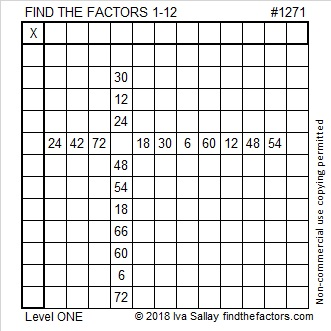# 1271 and Level 1

All the clues in this level 1 puzzle have a greatest common factor. If you can figure out what that GCF is, then you’ve completed the first step necessary to solve the puzzle.Print the puzzles or type the solution in this excel file: 12 factors 1271-1280

Now I’ll write a few facts about the number 1271:

• 1271 is a composite number.
• Prime factorization: 1271 = 31 × 41
• The exponents in the prime factorization are 1 and 1. Adding one to each and multiplying we get (1 + 1)(1 + 1) = 2 × 2 = 4. Therefore 1271 has exactly 4 factors.
• Factors of 1271: 1, 31, 41, 1271
• Factor pairs: 1271 = 1 × 1271 or 31 × 41
• 1271 has no square factors that allow its square root to be simplified. √1271 ≈ 35.651091271 is the sum of the nineteen prime numbers from 29 to 107.
It is also the sum of three consecutive primes: 419 + 421 + 431 = 1271

1271 is the hypotenuse of a Pythagorean triple:
279-1240-1271 which is 31 times (9-40-41)

This site uses Akismet to reduce spam. Learn how your comment data is processed.